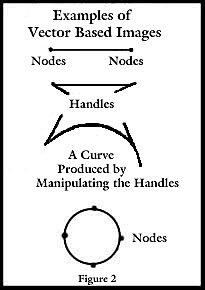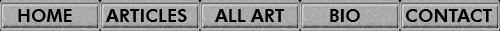Types of Images in the Field of Computer Graphics

While I wrote this article a few years ago for a class I was teaching, I have found people still refer to it. Thus, I am including it and updating it.

There are basically two types of graphic programs: those that are bitmap based and those that are vector based. The former comprise the  image editing and painting programs while the latter refer to the drawing programs. Programs such as Adobe Photoshop, Corel PhotoPaint and Painter, Macromedia Fireworks, etc. fall into the image editing and  painting category. CorelDraw, Adobe Illustrator, and Macromedia Freehand are examples of vector based drawing programs. Many programs, now, include tools for manipulating both types of images.A bitmap image is made up of pixels or bits (binary digits) of information arranged on a grid. Each bit can be visualized as a dot. The number of pixels per unit of measurement, example, ppi (pixels per inch) or dpi (dots per inch) determines the resolution of the image. These images are also referred to as raster images (and, somewhat confusingly, bitmap images). Simplistically, it could be stated that when one draws a line, one is covering pixels. See figure 1.Vector images are mathematical arrangements of points. These points are connected by mathematical formulae. Thus these images can be stretched and shrunk without changing the initial quality of the image. Almost all of the images are made by straight lines connected at nodes or points. However, one can create a curve that is absolutely smooth. The nodes can be manipulated to form smooth curves. The techniques involved in drawing programs differ markedly from those used in painting programs. See figure 2.

Since vector images are represented by mathematical formulae, they are resolution independent. This means that one can make a 1" x 1" square and stretch it to 5" x 5" without losing any image quality. The information is contained in the mathematical formula. If, however, one did that with the same 1" square in a raster based program, one would have to increase the number of pixels in the file. Since each pixel carries color or black and white information in it, the computer has to "decide" how to increase the number of pixels. This is one of the reasons why when one takes a web generated graphic at a screen resolution of 72 dpi and tries to increase the resolution to 200 dpi, for example, the colors can look awful. The computer had to decide the color of the pixels that it added. This technique of increasing the number of pixels in an image and keeping the linear dimensions, inches for example, of the object the same is called Resampling.

 Original File at a Resolution of 72 dpi Same File Resampled to a Resolution of 300 dpi width = 100 pixels width = 417 pixels height = 100 pixels height = 417 pixels linear width = 1.389 inches linear width = 1.389 inches linear height = 1.389 inches linear height = 1.389 inches file size = 30 KB file size = 510 KB

When the linear dimensions of a file are changed, that technique is called Resizing. For example, if one had a file that is 100 dpi and one increased the dimensions without adding pixels, the number of pixels or dots per inch, for example, would decrease. Conversely, if one decreased the linear dimensions of a file and did not remove pixels, the number of pixels or dots per inch would increase. Often, one uses combinations of the above.

 Original File at a Resolution of 72 dpi Same File Resized but NOT Resampled to a Resolution of 300 dpi width = 100 pixels width = 100 pixels height = 100 pixels height = 100 pixels linear width = 1.389 inches linear width = .333 inches linear height = 1.389 inches linear height = .333 inches file size 30 KB file size = 30 KB

In addition, the sizes of the files in a vector based program will always be much smaller than in a bitmap based program. Disregarding certain variables which are not important for this comparison, one could draw in a vector based program a 6" in diameter circle and fill it with a color. The file size would be 152KB (kilobytes). If one, then, exported the file as a TIFF file, which is a pixel based format, the size at screen resolution which is 72 dpi (the same as used for the web) would be 596 KB. If one had exported the file at 300 dpi, the size would have been 10MB (megabytes).